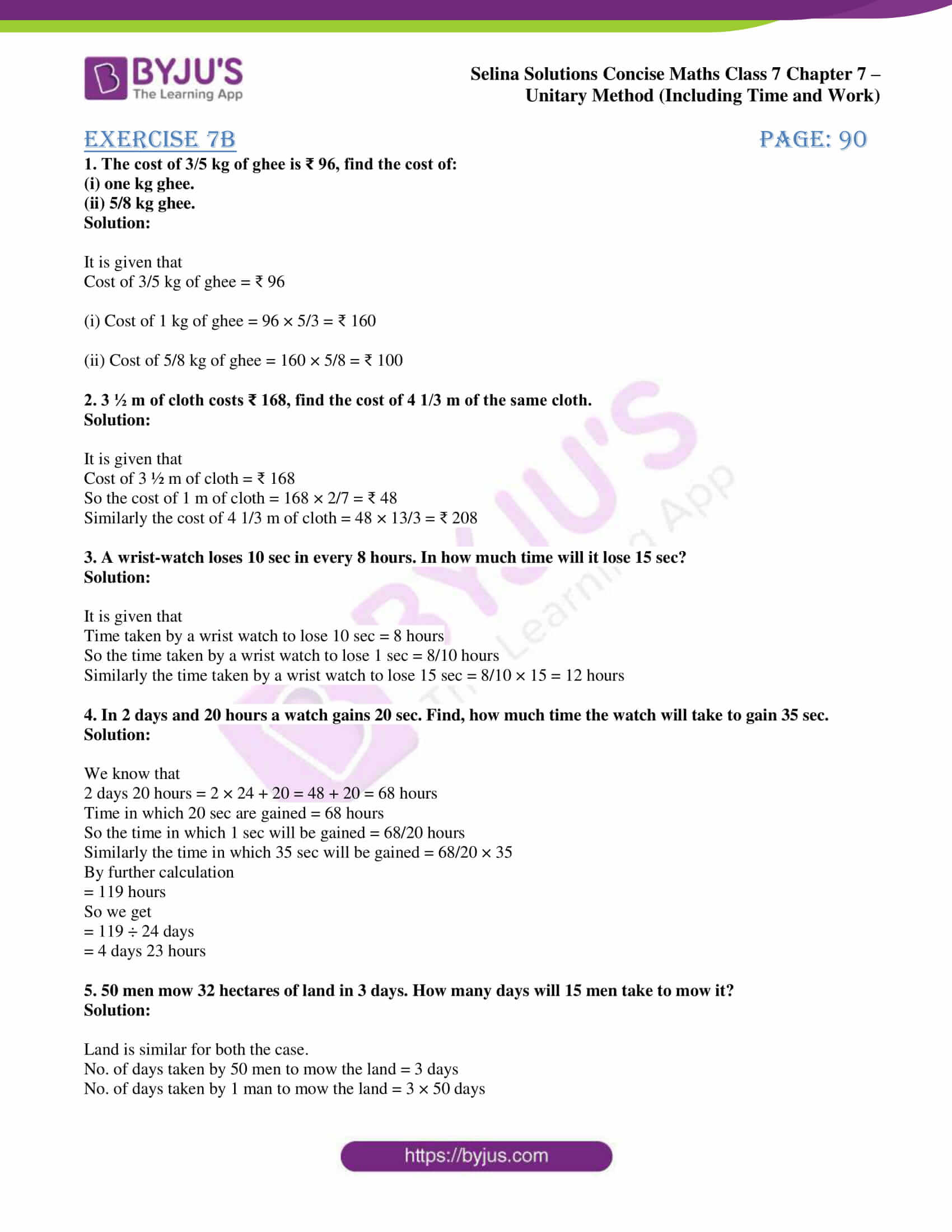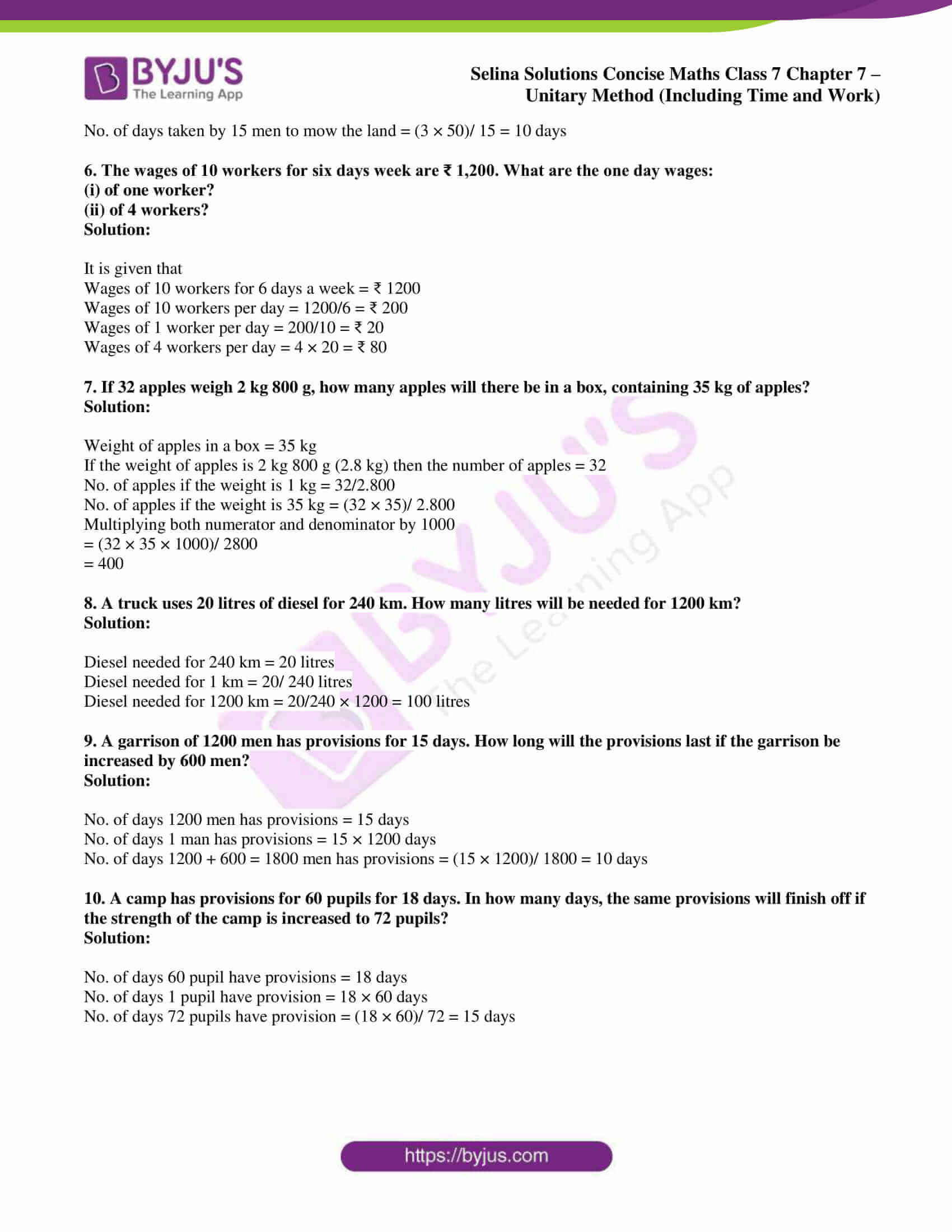# Selina Solutions Concise Maths Class 7 Chapter 7: Unitary Method (Including Time and Work) Exercise 7B

Selina Solutions Concise Maths Class 7 Chapter 7 Unitary Method (Including Time and Work) Exercise 7B provides students with the basic idea of solving tricky problems effortlessly. Students can download the PDF and solve the exercise wise problems at any point of time. The solutions are created in an interesting way to boost confidence among students in solving problems. For a clear knowledge about the concepts explained in this exercise, students can refer to Selina Solutions Concise Maths Class 7 Chapter 7 Unitary Method (Including Time and Work) Exercise 7B PDF, from the links which are provided here.

## Selina Solutions Concise Maths Class 7 Chapter 7: Unitary Method (Time and Work) Exercise 7B Download PDF### Access Selina Solutions Concise Maths Class 7 Chapter 7: Unitary Method (Time and Work) Exercise 7B

1. The cost of 3/5 kg of ghee is ₹ 96, find the cost of:

(i) one kg ghee.

(ii) 5/8 kg ghee.

Solution:

It is given that

Cost of 3/5 kg of ghee = ₹ 96

(i) Cost of 1 kg of ghee = 96 × 5/3 = ₹ 160

(ii) Cost of 5/8 kg of ghee = 160 × 5/8 = ₹ 100

2. 3 ½ m of cloth costs ₹ 168, find the cost of 4 1/3 m of the same cloth.

Solution:

It is given that

Cost of 3 ½ m of cloth = ₹ 168

So the cost of 1 m of cloth = 168 × 2/7 = ₹ 48

Similarly the cost of 4 1/3 m of cloth = 48 × 13/3 = ₹ 208

3. A wrist-watch loses 10 sec in every 8 hours. In how much time will it lose 15 sec?

Solution:

It is given that

Time taken by a wrist watch to lose 10 sec = 8 hours

So the time taken by a wrist watch to lose 1 sec = 8/10 hours

Similarly the time taken by a wrist watch to lose 15 sec = 8/10 × 15 = 12 hours

4. In 2 days and 20 hours a watch gains 20 sec. Find, how much time the watch will take to gain 35 sec.

Solution:

We know that

2 days 20 hours = 2 × 24 + 20 = 48 + 20 = 68 hours

Time in which 20 sec are gained = 68 hours

So the time in which 1 sec will be gained = 68/20 hours

Similarly the time in which 35 sec will be gained = 68/20 × 35

By further calculation

= 119 hours

So we get

= 119 ÷ 24 days

= 4 days 23 hours

5. 50 men mow 32 hectares of land in 3 days. How many days will 15 men take to mow it?

Solution:

Land is similar for both the case.

No. of days taken by 50 men to mow the land = 3 days

No. of days taken by 1 man to mow the land = 3 × 50 days

No. of days taken by 15 men to mow the land = (3 × 50)/ 15 = 10 days

6. The wages of 10 workers for six days week are ₹ 1,200. What are the one day wages:

(i) of one worker?

(ii) of 4 workers?

Solution:

It is given that

Wages of 10 workers for 6 days a week = ₹ 1200

Wages of 10 workers per day = 1200/6 = ₹ 200

Wages of 1 worker per day = 200/10 = ₹ 20

Wages of 4 workers per day = 4 × 20 = ₹ 80

7. If 32 apples weigh 2 kg 800 g, how many apples will there be in a box, containing 35 kg of apples?

Solution:

Weight of apples in a box = 35 kg

If the weight of apples is 2 kg 800 g (2.8 kg) then the number of apples = 32

No. of apples if the weight is 1 kg = 32/2.800

No. of apples if the weight is 35 kg = (32 × 35)/ 2.800

Multiplying both numerator and denominator by 1000

= (32 × 35 × 1000)/ 2800

= 400

8. A truck uses 20 litres of diesel for 240 km. How many litres will be needed for 1200 km?

Solution:

Diesel needed for 240 km = 20 litres

Diesel needed for 1 km = 20/ 240 litres

Diesel needed for 1200 km = 20/240 × 1200 = 100 litres

9. A garrison of 1200 men has provisions for 15 days. How long will the provisions last if the garrison be increased by 600 men?

Solution:

No. of days 1200 men has provisions = 15 days

No. of days 1 man has provisions = 15 × 1200 days

No. of days 1200 + 600 = 1800 men has provisions = (15 × 1200)/ 1800 = 10 days

10. A camp has provisions for 60 pupils for 18 days. In how many days, the same provisions will finish off if the strength of the camp is increased to 72 pupils?

Solution:

No. of days 60 pupil have provisions = 18 days

No. of days 1 pupil have provision = 18 × 60 days

No. of days 72 pupils have provision = (18 × 60)/ 72 = 15 days